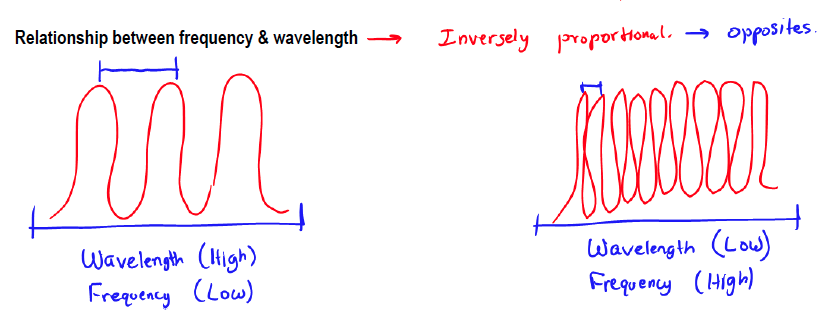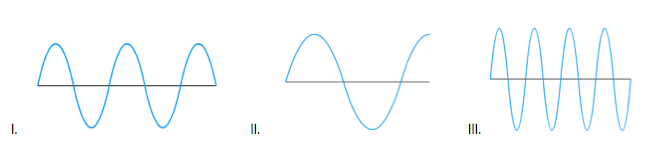Ch.7 - Quantum MechanicsWorksheetSee all chapters
 Ch.1 - Intro to General Chemistry 2hrs & 53mins 0% complete Worksheet Ch.2 - Atoms & Elements 2hrs & 49mins 0% complete Worksheet Ch.3 - Chemical Reactions 3hrs & 25mins 0% complete Worksheet BONUS: Lab Techniques and Procedures 1hr & 38mins 0% complete Worksheet BONUS: Mathematical Operations and Functions 47mins 0% complete Worksheet Ch.4 - Chemical Quantities & Aqueous Reactions 3hrs & 30mins 0% complete Worksheet Ch.5 - Gases 3hrs & 47mins 0% complete Worksheet Ch.6 - Thermochemistry 2hrs & 28mins 0% complete Worksheet Ch.7 - Quantum Mechanics 2hrs & 35mins 0% complete Worksheet Ch.8 - Periodic Properties of the Elements 1hr & 57mins 0% complete Worksheet Ch.9 - Bonding & Molecular Structure 2hrs & 5mins 0% complete Worksheet Ch.10 - Molecular Shapes & Valence Bond Theory 1hr & 31mins 0% complete Worksheet Ch.11 - Liquids, Solids & Intermolecular Forces 3hrs & 40mins 0% complete Worksheet Ch.12 - Solutions 2hrs & 17mins 0% complete Worksheet Ch.13 - Chemical Kinetics 2hrs & 22mins 0% complete Worksheet Ch.14 - Chemical Equilibrium 2hrs & 26mins 0% complete Worksheet Ch.15 - Acid and Base Equilibrium 4hrs & 42mins 0% complete Worksheet Ch.16 - Aqueous Equilibrium 3hrs & 48mins 0% complete Worksheet Ch. 17 - Chemical Thermodynamics 1hr & 44mins 0% complete Worksheet Ch.18 - Electrochemistry 2hrs & 58mins 0% complete Worksheet Ch.19 - Nuclear Chemistry 1hr & 33mins 0% complete Worksheet Ch.20 - Organic Chemistry 3hrs 0% complete Worksheet Ch.22 - Chemistry of the Nonmetals 2hrs & 1min 0% complete Worksheet Ch.23 - Transition Metals and Coordination Compounds 1hr & 54mins 0% complete Worksheet

# Wavelength and Frequency

See all sections

Wavelength is the distance from one crest of a wave to another, whereas frequency is the number of waves within a second.

###### The Electromagnetic Spectrum

The electromagnetic spectrum consists of varying forms of energy within oscillating electric and magnetic fields.

Concept #1: The Electromagnetic Spectrum

Transcript

Welcome back guys. In this new video, we're going to take a look at the nature of light. Now, what we should realize is the visible light that we see with our eyes, it only represents a small section of all the possible lights that exists. The visible light that we see has a certain energy tied with it.
Now, there are light sources that are above that energy and below that energy. Taking into account all of these different types of energies, we have this image right here. This is known as our electromagnetic spectrum. You should see that we have here, these are gamma rays, we have X-rays, UV, here this is infrared, IR.
Now, between UV which is ultraviolet light and IR, which is infrared light, that's what we find our visible light spectrum. We're going to say our visible light spectrum—the range is really 390 nm to about 780 nm. We learned this in the school, roygbiv, so roygbiv. Remember, what is that stand for? We're going to say red, orange, yellow, green, blue, indigo and violet.
We're going to say here, it looks like violet, violet is around 400 nm, whereas red is around 780 nm. Remember, the visible light spectrum only represent a small section of the entire spectrum of colors. Now, after IR, there are these microwave, then we have radio waves, which we get in our car radios or at home, on our stereos. We have FM and then we have radio in between AM and then we have long radio waves.
These represent basically all the different types of light sources or light energies that exist. There is also a cosmic rays which are usually above gamma, the professors usually ignore those.

Concept #2: Wavelength & Frequency

Transcript

We're going to say according to this graph, there are basically wave properties associated with this electromagnetic spectrum.
We're going to say there are two independent variables associated with this electromagnetic spectrum. We're going to say the first one uses the variable V which really stands for mu, the Greek mu. In it is the number of waves you have per second, that right there would be our frequency. It is expressed in units of seconds inverse or hertz. Just remember, seconds inverse or hertz mean the same exact thing.
The second variable associated with our electromagnetic spectrum is lambda. This represents the distance from one quest of a wave to the other and this is our wavelength. The units for wavelength are meters. We're going to say that in our electromagnetic spectrum, we have frequency and we have wavelength.

Concept #3: The relationship between Wavelength & Frequency

Transcript

As you can see, we're going to say, it looks like long radio waves, they have the highest wavelength, because they're above 108 meters, but as a result their frequency is very low, so they would have the lowest frequency. On the other side, it looks like gamma rays is 10/24 for frequency, so these guys would have the highest frequency and on the bottom, therefore it'd have the lowest wavelength.
Looking out what happens to our frequency when our wavelength is affected. Remember, we've talked about this before, we would say that the relationship between frequency and wavelength is that they are inversely proportional, which means they're opposite of one another. The way you should understand it visually is like this, so we have light travels sometimes as waves according to some theories. Here are some waves. These represent a bunch of light particles together.
Now, what we're going to say here is that frequency is how many waves do you get per second? Then, here we're going to say that wavelength is the distance from one top of the wave to the other. In this image here, we'd say that, the distance is pretty big, so we'd say that the wavelength is high, but we only get three waves in a second. That's not that many. We'd say that our frequency is low.
Now, if we draw a different picture, this is roughly the same distance as the first set of waves that I've showed you, but here now, you would say that the distance from one top of the wave to the other decrease. The wavelength looks like it's very small, so the wavelength would be low. We also say that we get a lot more waves per second, for that period of time, so we'd say that frequency here is high.
Visually, this is how you would show that frequency and wavelength are inversely proportional. Meaning that they are opposite of one another. We've talked about this when we talked about the relationship between certain factors such as pressure and volume. They're inversely proportional to each other. Same way, wavelength and frequency are inversely proportional.
Now, what this have to do with our electromagnetic spectrum? Our electromagnetic spectrum is a way of associating light with energy. We're going to say here, highest frequency, frequency has a direct relationship to energy. We'd say that gamma rays according to this spectrum have the highest frequency and therefore they have the highest energy. So they're both directly related to each other.
Remember, wavelength and frequency are opposites of one another. If my energy is high, therefore my wavelength would be low. On this other side, because my frequency is low, that means my energy is low.
It's going to become important that you guys remember the order of these different types of light sources, because your professor will easily ask one of them on the exam, 'Rank these in terms of increasing energy. Rank these in terms of increasing frequency.' It becomes important to remember the order.

Wavelength and Frequency are inversely proportional, meaning that if one increases then the other one must decrease.Practice: A. Based on the images of different electromagnetic waves, answer each of the following questions.

a) Which electromagnetic wave has the longest wavelength?

b) Which electromagnetic wave has the greatest energy?

c) Which electromagnetic wave has the lowest frequency?

d) Which electromagnetic wave has the largest amplitude?

###### Wavelength & Frequency Calculations

Concept #4: Converting between Wavelength and   Frequency

Transcript

In this new video, we're going to continue our discussions of the relationship between frequency and wavelength and see how do we mathematically go from one to the other. We're going to say that the speed of a wave or speed of a light wave is the product of the frequency times the wavelength or between mu
times lambda. We're going to say in a vacuum, all forms of radiation travel at a constant 3.00 times 10 to the 8 meters per second. This is a physical constant that's known as the speed of light. We're going to say the speed of light just equals our wavelength times our frequency.
Basically, we're going to look at this equation right here. With this his equation, we’re able to go from frequency to wavelength because C is always that number, 3.00 times 10 to the 8 meters per second.

To convert between wavelength & frequency we use the equation c = ν  λ, where c equals the speed of light.

Example #1: Even the music we listen to deals with how energy travels to get to our car radio. If Power 96 broadcasts its music at 96.5 MHz (megahertz, or 106 Hertz) find the wavelength in micrometers and Angstroms of the radio waves.

Practice: Calculate the frequency of the red light emitted by a neon sign with a wavelength of 663.8 nm.